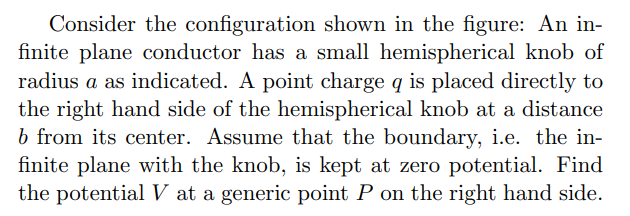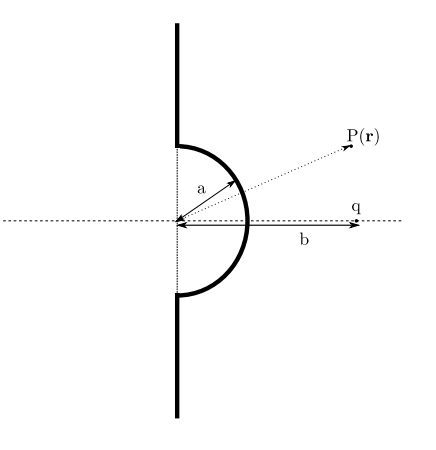# Consider the configuration shown in the figure: An in- finite plane conductor has a small hemispherical knob of radius a as indicated. A point charge q is placed directly to the right hand side of the hemispherical knob at a distance b from its center. Assume that the boundary, i.e. the in- finite plane with the knob, is kept at zero potential. Find the potential V at a generic point P on the right hand side. P(r) b

Questionhelp_outlineImage TranscriptioncloseConsider the configuration shown in the figure: An in- finite plane conductor has a small hemispherical knob of radius a as indicated. A point charge q is placed directly to the right hand side of the hemispherical knob at a distance b from its center. Assume that the boundary, i.e. the in- finite plane with the knob, is kept at zero potential. Find the potential V at a generic point P on the right hand side. fullscreenhelp_outlineImage TranscriptioncloseP(r) b fullscreen

### Want to see the step-by-step answer?